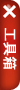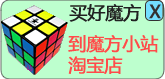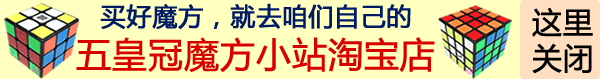调整角块朝向的公式 角块朝向的 第1招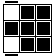(R U R' U R U2 R') (L' U' L U' L' U'2 L)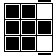(L' U' L U' L' U'2 L)(R U R' U R U2 R') 就是上面那个公式两个括号倒一下。 角块朝向的 第2招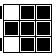(L' U2 L U L' U L) (R U'2 R' U' R U' R') 就是第1招第一个公式的逆公式。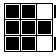(R U'2 R' U' R U' R')(L' U2 L U L' U L) 也是把上一个公式两个括号倒一下。 角块朝向的 第3招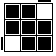z' (R U R' U')2 L2 (U R U' R')2 L2 z z’就是让黄色面冲左 z就是把黄色面转回到顶面 (R U R' U')2的意思就是R U R' U'做两次 这种情况就是右上那个角要逆时针转，就把黄色面冲左，然后先做(R U R' U')2，然后左边转180度，再做 (U R U' R')2，然后左边再转180度恢复左面，然后记得把魔方整体转回来，让黄色面重新朝上。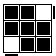z' (U R U' R')2 L2 (R U R' U')2 L2 z 这种情况就是右上那个角要顺时针转，就把黄色面冲左，然后先做 (U R U' R')2，然后左边转180度，再做(R U R' U')2，然后左边再转180度恢复左面，然后记得把魔方整体转回来，让黄色面重新朝上。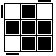z' ((R U R' U')2 L)3 L z ((R U R' U')2 L)3 的意思就是 (R U R' U')*2 L 做三次 右上这个角要逆时针转，就用 (R U R' U')2 记得在这个公式里左面顺着一共转了360°，在所有第3招的情况里，左边都是同一方向一共转了360°。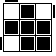z' ((U R U' R')2 L)3 L z 右上这个角要顺时针转，就用 (U R U' R')2 两个小鱼另外的解法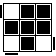(R U' U' R' U' R U' R') U (R2' U)(R U R' U')(R' U')(R' U R') U 注意这个小鱼初始的位置和上面的小鱼是不同的。这个公式就是这个形状的OLL公式+U+顺时针三棱换PLL公式+U(R' U2 R U R' U R) U (R U ' R) U (R U R U') (R' U' R2) U 就是这个形状的OLL公式+U+逆时针三棱换PLL公式+U 角块朝向的第3招在底面用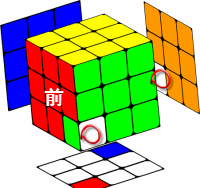(R U R' U')2 D2 (U R U' R')2 D2 底面两个角一顺一逆，其中靠近你的右前角是顺时针。这时不用翻转魔方让黄色面冲左，直接用(R U R' U')2（注意这里右前这个角是顺时针时是先用(R U R' U')2），然后转底面180度，再 (U R U' R')2，然后再D2恢复底面。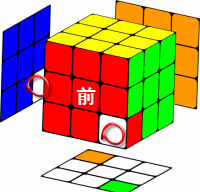(U R U' R')2 D2 (R U R' U')2 D2 底面两个角一逆一顺，其中靠近你的右前角是逆时针。这时先用(U R U' R')2（注意这里右前这个角是逆时针时是先用(U R U' R')2），然后转底面180度，再 (R U R' U')2，然后再D2恢复底面。 角块朝向两个提高速度的公式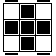(R U U) (R' U' R U R' U') (R U' R') U2 (R'2 U)(R U R' U')(R' U')(R' U R') U2 等于就是这个形态的OLL公式+U2+顺时针三棱换PLL公式+U2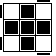R U'U' (R'2 U') (R2 U') R'2 U2 R (R U ' R) U (R U R U') (R' U' R2) U2 等于就是这个形态的OLL公式+逆时针三棱换PLL公式+U2 调整棱块朝向的公式 棱块朝向第1招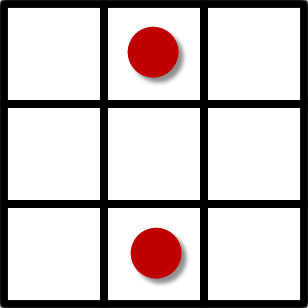顶层对棱翻 (M'U)2 M' U2 (M U)2 M U2 其中M’的意思就是中层向上一下 M的意思是中层向下一下 也就是上顺上顺上顺顺，下顺下顺下顺顺 棱块朝向第2招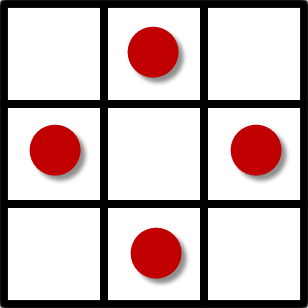顶层四棱翻 (M'U)4 (MU)4 其中M’的意思就是中层向上一下 M的意思是中层向下一下 也就是四个上顺+四个下顺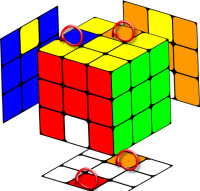顶层左上相邻的两个+ 底层前后相对两个一起翻 (M'U)4 其中M’的意思就是中层向上一下 也就是四个上顺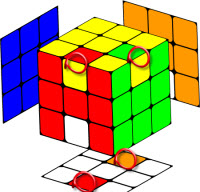顶层右下相邻的两个+ 底层前后相对两个一起翻 (MU)4 其中M的意思就是中层向下一下 也就是四个下顺 棱块朝向第3招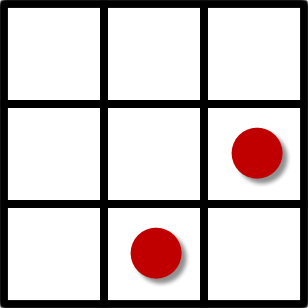顶层右下相邻两个棱翻 (R' U2) (R'2 U R' U')(R' U2) (r U R U')r' 其中r的意思是右侧两层顺时针转，r'的意思是右侧两层逆时针转如果上面这个公式忘了，你也可以用对棱翻+准备回复的策略把这个情况搞定，如右所示。 (R B)(M'U)2 M' U2 (M U)2 M U2 (B' R') 就是准备(R B)把右边这个块准备到对棱的位置，然后上顺上顺上顺顺，下顺下顺下顺顺，然后(B' R')回复。 调整棱块顺序公式 两个PLL三棱换公式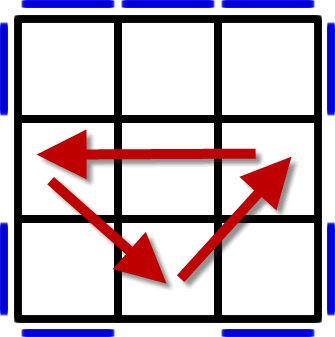(R U ' R) U (R U R U') (R' U' R2) 有下划线的U'是用左手食指，斜体的 U' 或者 F' 是用右手拇指。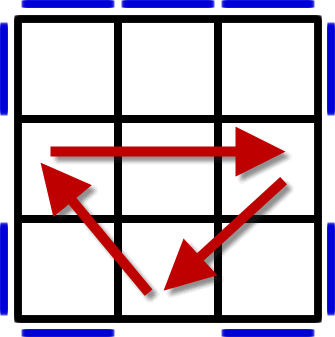(R'2 U)(R U R' U') (R' U')(R' U R') 调整角块顺序公式 两个PLL三角换公式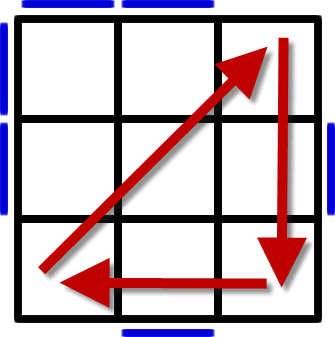x' R2 D2(R' U' R) D2(R' U R') x x'表示魔方整体旋转，方向就是让黄色面冲着你自己。有下划线的U'是用左手食指，斜体的 U' 或者 F' 是用右手拇指。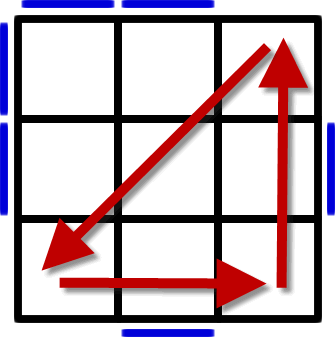x'(R U'R) D2 (R'U R) D2 R2 x x'表示魔方整体旋转，方向就是让黄色面冲着你自己。有下划线的U'是用左手食指，斜体的 U' 或者 F' 是用右手拇指。 第二个公式，三个角块的三轮换。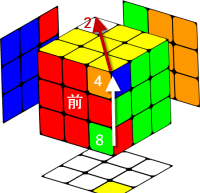第一个解法： (R' F'R2 F R U2)2 看8这个角是往上走，就用正公式。他是 8到4, 4到2, 2到8 的一个三轮换。 第二个解法： ((R' F R F')3 U2)2 就是(R' F R F')做三次，然后加上U2，然后上面步骤重复一遍。这个公式长一些，但更好记。 解法1： 解法2：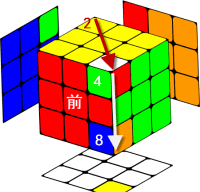第一个解法： (U2 R' F'R2 F R )2 看4这个角是往下走，就用逆公式。他是 2到4, 4到8, 8到2 的一个三轮换。 第二个解法： (U2 (R' F R F')3 )2 解法1： 解法2：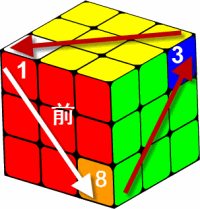第一个解法： U (R'F'R2 FRU2) (R'F'R2 FR) U 这个公式就是用了一个准备回复的策略，加上上面的正公式。本来最后应该是U2+U',最后就等同于一个U。 第二个解法： U(R'FR F')3 U2 (R'FR F')3 U 跟第一个解法的道理一样，本来最后应该是U2+U',最后就等同于一个U。 解法1： 解法2：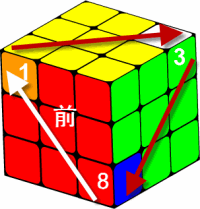第一个解法： U' (R'F'R2 FRU2) (R'F'R2 FR) U' 这个公式同样是用了一个准备回复的策略，加上上面的正公式。本来最后应该是U2+U,最后就等同于一个U'。 第二个解法： U'(R'FR F')3 U2 (R'FR F')3 U' 跟第一个解法的道理一样，本来最后应该是U2+U,最后就等同于一个U'。 解法1： 解法2：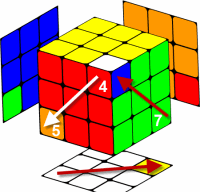(x2 y) U(R'F'R2 FRU2)(R'F'R2 FR) U (y' x'2) 这个公式就是翻了一下魔方，做前面8到3到1再回到8的公式，U (R'F'R2 FRU2) (R'F'R2 FR) U， (x2 y)就是把魔方翻过来白色面朝上同时让红色面朝右。 ，最后(y' x'2)再把魔方翻回来。 第三个公式，三个角块的三轮换。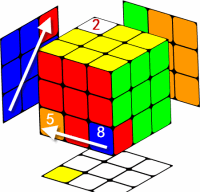(L2 U R2 U')2 看5这个角是往上走，就用正公式。他是 5到2, 2到8, 8到5 的一个三轮换。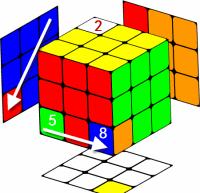(U R2 U' L2)2 看2这个角是往下走，就用逆公式。他是 2到5, 5到8, 8到2 的一个三轮换。 奇偶校验PLL公式 1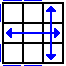(R U R' U')(R' F)(R2 U' R' U')(R U R' F') 有下划线的U'是用左手食指，斜体的 U' 或者 F' 是用右手拇指。 3D动画 2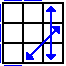(R U R' F')(R U R' U')(R' F)(R2 U' R' U') 3D动画 3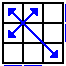F(R U'R' U')(R U R' F')(R U R' U')(R' F R F') 3D动画 4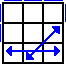z(U' R D')(R2 U R' U' R2 U) D R' 3D动画 5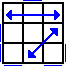(R' U2)(R U'U')(R' F R U R' U')(R'F' R2 U') 3D动画 6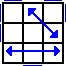(R U'U')(R' U2)(R B ' R' U')(R U R B R2' U) 3D动画 7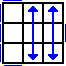U' (R'U R U') R2' b' x (R' U R) y' (R U R' U' R2) 3D动画 8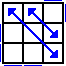(R' U R' d') (R' F') (R2 U' R' U)(R' F R F) 3D动画 9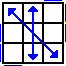z(R' U R')z'(R U2 L' U R') z(U R')z'(R U2 L' U R') 3D动画 10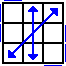z (U'R D') (R2 U R'U') z' (R U R') z (R2 U R') D R' 3D动画

#### 您可以直接写信给我，任何的只言片语的建议感想都可以的：）我会尽量回复大家。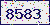验证码看不清楚?请点击验证码图片获得一个新的。